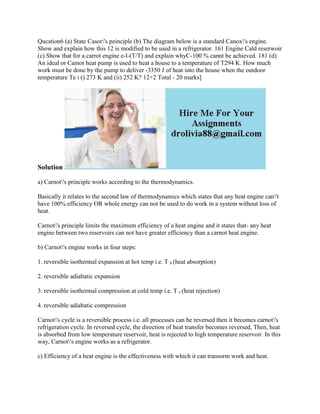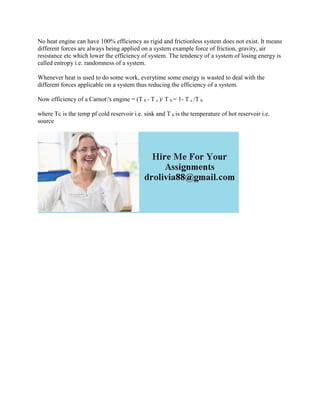Diese Präsentation wurde erfolgreich gemeldet.

# Qucstion6 (a) State Casor-'s peinciple (b) The diagram below is a stan.docx

Anzeige
Anzeige
Anzeige
Anzeige
Anzeige
Anzeige
Anzeige
Anzeige
Anzeige
Anzeige
Anzeige×

1 von 2 Anzeige

# Qucstion6 (a) State Casor-'s peinciple (b) The diagram below is a stan.docx

Qucstion6 (a) State Casor\'s peinciple (b) The diagram below is a standard Canox\'s engine. Show and explain how this 12 is modified to be used in a refrigerator. 161 Engine Cald reserwoir (c) Show that for a carrot engine e-l-(T/T) and explain whyC-100 % cannt be achieved. 181 (d) An ideal or Camot heat pump is used to heat a house to a temperature of T294 K. How much work must be done by the pump to deliver -3350 J of heat into the house when the outdoor temperature Te i () 273 K and (ii) 252 K? 12+2 Total - 20 marks]
Solution
a) Carnot\'s principle works according to the thermodynamics.
Basically it relates to the second law of thermodynamics which states that any heat engine can\'t have 100% efficiency OR whole energy can not be used to do work in a system without loss of heat.
Carnot\'s principle limits the maximum efficiency of a heat engine and it states that- any heat engine between two reservoirs can not have greater efficiency than a carnot heat engine.
b) Carnot\'s engine works in four steps:
1. reversible isothermal expansion at hot temp i.e. T h (heat absorption)
3. reversible isothermal compression at cold temp i.e. T c (heat rejection)
Carnot\'s cycle is a reversible process i.e. all processes can be reversed then it becomes carnot\'s refrigeration cycle. In reversed cycle, the direction of heat transfer becomes reversed, Then, heat is absorbed from low temperature reservoir, heat is rejected to high temperature reservoir. In this way, Carnot\'s engine works as a refrigerator.
c) Efficiency of a heat engine is the effectiveness with which it can transorm work and heat.
No heat engine can have 100% efficiency as rigid and frictionless system does not exist. It means different forces are always being applied on a system example force of friction, gravity, air resistance etc which lower the efficiency of system. The tendency of a system of losing energy is called entropy i.e. randomness of a system.
Whenever heat is used to do some work, everytime some energy is wasted to deal with the different forces applicable on a system thus reducing the efficiency of a system.
Now efficiency of a Carnot\'s engine = (T h - T c )/ T h = 1- T c /T h
where Tc is the temp pf cold reservoir i.e. sink and T h is the temperature of hot reservoir i.e. source
.

Qucstion6 (a) State Casor\'s peinciple (b) The diagram below is a standard Canox\'s engine. Show and explain how this 12 is modified to be used in a refrigerator. 161 Engine Cald reserwoir (c) Show that for a carrot engine e-l-(T/T) and explain whyC-100 % cannt be achieved. 181 (d) An ideal or Camot heat pump is used to heat a house to a temperature of T294 K. How much work must be done by the pump to deliver -3350 J of heat into the house when the outdoor temperature Te i () 273 K and (ii) 252 K? 12+2 Total - 20 marks]
Solution
a) Carnot\'s principle works according to the thermodynamics.
Basically it relates to the second law of thermodynamics which states that any heat engine can\'t have 100% efficiency OR whole energy can not be used to do work in a system without loss of heat.
Carnot\'s principle limits the maximum efficiency of a heat engine and it states that- any heat engine between two reservoirs can not have greater efficiency than a carnot heat engine.
b) Carnot\'s engine works in four steps:
1. reversible isothermal expansion at hot temp i.e. T h (heat absorption)
3. reversible isothermal compression at cold temp i.e. T c (heat rejection)
Carnot\'s cycle is a reversible process i.e. all processes can be reversed then it becomes carnot\'s refrigeration cycle. In reversed cycle, the direction of heat transfer becomes reversed, Then, heat is absorbed from low temperature reservoir, heat is rejected to high temperature reservoir. In this way, Carnot\'s engine works as a refrigerator.
c) Efficiency of a heat engine is the effectiveness with which it can transorm work and heat.
No heat engine can have 100% efficiency as rigid and frictionless system does not exist. It means different forces are always being applied on a system example force of friction, gravity, air resistance etc which lower the efficiency of system. The tendency of a system of losing energy is called entropy i.e. randomness of a system.
Whenever heat is used to do some work, everytime some energy is wasted to deal with the different forces applicable on a system thus reducing the efficiency of a system.
Now efficiency of a Carnot\'s engine = (T h - T c )/ T h = 1- T c /T h
where Tc is the temp pf cold reservoir i.e. sink and T h is the temperature of hot reservoir i.e. source
.

Anzeige
Anzeige

### Qucstion6 (a) State Casor-'s peinciple (b) The diagram below is a stan.docx

1. 1. Qucstion6 (a) State Casor's peinciple (b) The diagram below is a standard Canox's engine. Show and explain how this 12 is modified to be used in a refrigerator. 161 Engine Cald reserwoir (c) Show that for a carrot engine e-l-(T/T) and explain whyC-100 % cannt be achieved. 181 (d) An ideal or Camot heat pump is used to heat a house to a temperature of T294 K. How much work must be done by the pump to deliver -3350 J of heat into the house when the outdoor temperature Te i () 273 K and (ii) 252 K? 12+2 Total - 20 marks] Solution a) Carnot's principle works according to the thermodynamics. Basically it relates to the second law of thermodynamics which states that any heat engine can't have 100% efficiency OR whole energy can not be used to do work in a system without loss of heat. Carnot's principle limits the maximum efficiency of a heat engine and it states that- any heat engine between two reservoirs can not have greater efficiency than a carnot heat engine. b) Carnot's engine works in four steps: 1. reversible isothermal expansion at hot temp i.e. T h (heat absorption) 2. reversible adiabatic expansion 3. reversible isothermal compression at cold temp i.e. T c (heat rejection) 4. reversible adiabatic compression Carnot's cycle is a reversible process i.e. all processes can be reversed then it becomes carnot's refrigeration cycle. In reversed cycle, the direction of heat transfer becomes reversed, Then, heat is absorbed from low temperature reservoir, heat is rejected to high temperature reservoir. In this way, Carnot's engine works as a refrigerator. c) Efficiency of a heat engine is the effectiveness with which it can transorm work and heat.
2. 2. No heat engine can have 100% efficiency as rigid and frictionless system does not exist. It means different forces are always being applied on a system example force of friction, gravity, air resistance etc which lower the efficiency of system. The tendency of a system of losing energy is called entropy i.e. randomness of a system. Whenever heat is used to do some work, everytime some energy is wasted to deal with the different forces applicable on a system thus reducing the efficiency of a system. Now efficiency of a Carnot's engine = (T h - T c )/ T h = 1- T c /T h where Tc is the temp pf cold reservoir i.e. sink and T h is the temperature of hot reservoir i.e. source